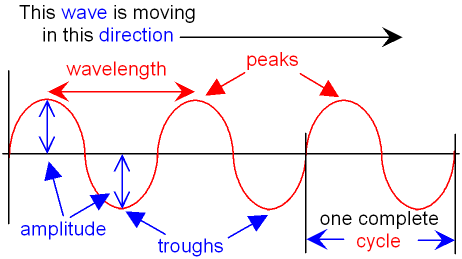gcsescience.com                                       4                                       gcsescience.com

Waves

Transverse Waves (continued).

Below is a picture of a transverse wave.What is the Wavelength of a Transverse Wave?

The wavelength of a transverse wave is the
distance between two peaks or the distance between two troughs.
Wavelength can be defined as
"the distance the wave has traveled during one complete cycle".
Wavelength is given the symbol λ.
This is the Greek letter lambda, pronounced lam-der.
Wavelength is measured in metres because it is a distance.

What is the Frequency of a Wave?

Frequency is defined as
"the number of complete cycles (complete waves) in one second".
Hertz is the unit of frequency (symbol Hz).
1 hertz = 1 cycle per second.

What is the Period of a Wave?

The period of a wave is defined as
"the time taken for one complete cycle".

The period = 1 ÷ frequency.

This can be rearranged to give
Frequency = 1 ÷ period.

What is the Amplitude of a Transverse Wave?

The amplitude of a transverse wave
is measured from the peak (or trough) to the mid-point.
Amplitude can be defined as
"the maximum displacement from the average position".
Amplitude is a measure of how much energy the wave has.

gcsescience.com       Physics Quiz       Index       Wave Quiz       gcsescience.com